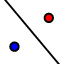# Reflecting About Lines

## DIRECTIONS:

1) Position Wily Coyote (using points A and B) anywhere you'd like within the coordinate plane. 2) Select the REFLECT ABOUT LINEtool. Then drag a rectangle around points A, B, and Wily's picture. This should cause these 3 items to become highlighted. Select the dashed line. 3) The images of these items should now appear. Please answer the questions that appear below the applet.

## 1.

If point A is (1,-2) and point B is (4,-1), what would point A' and point B' be if you reflect across the line y=x.

## 2.

What changed with point A and point B when you reflected over the line y=x to get point A' and point B'?